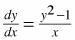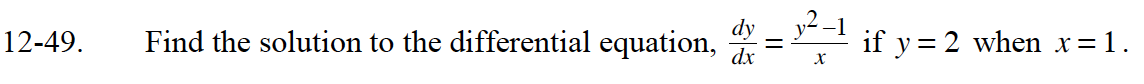### Home > CALC > Chapter 12 > Lesson 12.1.4 > Problem12-49

12-49.

Find the solution to the differential equation,if y = 2 when x = 1. Homework Help ✎$A=-\frac{1}{2}, B=\frac{1}{2}$

$\int\Big(\frac{1}{-2(y+1)}+\frac{1}{2(y-1)}\Big)dy=\int\frac{1}{x}dx$

$-\frac{1}{2}\ln|y+1|+\frac{1}{2}\ln|y-1|=\ln|x|+C$

$\ln\Bigg|\frac{y-1}{y+1}\Bigg|=2\ln|x|+C$

$\frac{y-1}{y+1}=Cx^2$

Use x = 1 and y = 2 to solve for C.
Then use the value of C and algebra to write the solution in y = form.